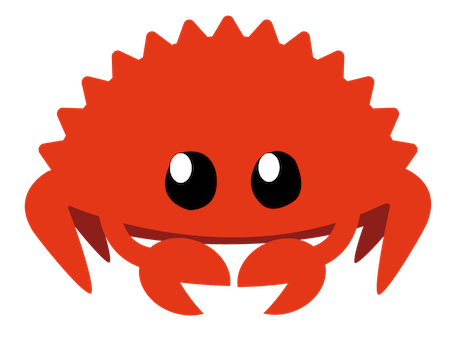# Crate funspace

Expand description

## FunspaceCollection of function spaces.

## Bases

ChebyshevR2rTrueNonechebyshev()
ChebDirichlet R2rFalseu(-1) = u(1) = 0cheb_dirichlet()
ChebNeumannR2rFalseu’(-1) = u’(1) = 0cheb_neumann()
ChebDirichletNeumannR2rFalseu(-1) = u’(1) = 0cheb_dirichlet_neumann()
ChebBiHarmonicAR2rFalseu(-1) = u’(-1) = u(1)= u’(1) = 0cheb_biharmonic_a()
ChebBiHarmonicBR2rFalseu(-1) = u’‘(-1) = u(1)= u’’(1) = 0cheb_biharmonic_b()
FourierR2cR2cTruePeriodicfourier_r2c()
FourierC2cC2cTruePeriodicfourier_c2c()

### Transform

A transformation describes a conversion from physical values to spectral coefficients, and vice versa. A fourier transform, for example, of a function gives complex-valued coefficients representing the sinusoid functions. A chebyshev transform gives real-valued spectral coefficients, representing Chebyshev polynomials. The transformations are implemented by the BaseTransform trait.

#### Example

Apply forward transform of 1d array in cheb_dirichlet space

use funspace::traits::BaseTransform;
use funspace::cheb_dirichlet;
use ndarray::{Array1, array};
let mut cd = cheb_dirichlet::<f64>(5);
let input = array![1., 2., 3., 4., 5.];
let output: Array1<f64> = cd.forward(&input, 0);

### Differentiation

Differentiation in spectral space is generally efficient and very accurate. Differentiation in Fourier space becomes a simple multiplication of the spectral coefficients with the wavenumber vector. Differentiation in Chebyshev space is done by a recurrence relation and almost as fast as in Fourier space. Each base implements a differentiation method, which is applied to an array of coefficents. This is defined by the BaseGradient trait.

#### Example

Differentiation

use funspace::fourier_r2c;
use ndarray::Array1;
use num_complex::Complex;
// Define base
let mut fo = fourier_r2c(8);
// Get coordinates in physical space
let x: Vec<f64> = fo.coords().clone();
let v: Array1<f64> = x
.iter()
.map(|x| (2. * x).sin())
.collect::<Vec<f64>>()
.into();
// Transform to physical space
let vhat: Array1<Complex<f64>> = fo.forward(&v, 0);

// Apply differentiation twice along first axis
let dvhat = fo.gradient(&vhat, 2, 0);
// Transform back to spectral space
let dv: Array1<f64> = fo.backward(&dvhat, 0);
// Compare with correct derivative
for (exp, ist) in x
.iter()
.map(|x| -4. * (2. * x).sin())
.collect::<Vec<f64>>()
.iter()
.zip(dv.iter())
{
assert!((exp - ist).abs() < 1e-5);
}

### Orthogonal and composite Bases

Function spaces such as those of Fourier polynomials or Chebyshev polynomials are are considered orthogonal, i.e. the dot product of every single polynomial with any other polynomial in its set vanishes. In this cases the mass matrix is a purely diagonal matrix. However, other function spaces can be constructed by a linear combination of the orthogonal basis functions, i.e. they are composite bases. In this way, function spaces can be constructed that satisfy certain boundary conditions such as Dirichlet (u(x0) = 0) or Neumann (u’(x0) = 0). This is used to solve partial differential equations (see Galerkin method).

To switch from its composite form to the orthogonal form, each base implements a BaseFromOrtho trait, which defines the transform to_ortho and from_ortho. If the base is already orthogonal, the input will be returned, otherwise it is transformed from the composite space to the orthogonal space (to_ortho), or vice versa (from_ortho). Note that the size of the composite space m is usually less than its orthogonal counterpart n, i.e. the spectral representation has less coefficients. In other words, the composite space is a lower dimensional subspace of the orthogonal space.

#### Example

Transform composite space cheb_dirichlet to its orthogonal counterpart chebyshev. Note that cheb_dirichlet has 6 spectral coefficients, while the chebyshev bases has 8.

use funspace::traits::{BaseTransform, BaseElements, BaseFromOrtho};
use funspace::{cheb_dirichlet, chebyshev};
use std::f64::consts::PI;
use ndarray::prelude::*;
use ndarray::Array1;
use num_complex::Complex;
// Define base
let ch = chebyshev(8);
let cd = cheb_dirichlet(8);
// Get coordinates
let x: Vec<f64> = ch.coords().clone();
let v: Array1<f64> = x
.iter()
.map(|x| (PI / 2. * x).cos())
.collect::<Vec<f64>>()
.into();
// Transform to spectral space
let ch_vhat: Array1<f64> = ch.forward(&v, 0);
let cd_vhat: Array1<f64> = cd.forward(&v, 0);
// Send array to orthogonal space (cheb_dirichlet -> chebyshev)
let cd_vhat_ortho = cd.to_ortho(&cd_vhat, 0);
// Both arrays are equal, because field was
// initialized with dirichlet boundary conditions.
for (exp, ist) in ch_vhat.iter().zip(cd_vhat_ortho.iter()) {
assert!((exp - ist).abs() < 1e-5);
}

// However, if the function values do not satisfy
// dirichlet boundary conditions, they will
// be enforced by the transform to the cheb_dirichlet function
// space, thus the transformed values will deviate
// from a pure chebyshev transform (which does not
// enfore the boundary conditions).
let mut v: Array1<f64> = x
.iter()
.map(|x| (PI / 2. * x).sin())
.collect::<Vec<f64>>()
.into();
let ch_vhat: Array1<f64> = ch.forward(&v, 0);
let cd_vhat: Array1<f64> = cd.forward(&v, 0);
let cd_vhat_ortho = cd.to_ortho(&cd_vhat, 0);
// They will deviate
println!("chebyshev     : {:?}", ch_vhat);
println!("cheb_dirichlet: {:?}", cd_vhat_ortho);

### MPI Support (Feature)

Funspace comes with limited mpi support. Currently this is restricted to 2D spaces. Under the hood it uses a fork of the rust mpi libary https://github.com/rsmpi/rsmpi which requires an existing MPI implementation and libclang.

Activate the feature in your Cargo.toml

funspace = {version = "0.4", features = ["mpi"]}

#### Examples

examples/space_mpi.rs

Install cargo mpirun, then

cargo mpirun --np 2 --example space_mpi --features="mpi"

## Versions

• v0.4.0: Major API changes + New methods
• v0.3.0: Major API changes + Performance improvements

## Re-exports

pub use crate::enums::BaseC2c;
pub use crate::enums::BaseKind;
pub use crate::enums::BaseR2c;
pub use crate::enums::BaseR2r;
pub use space::traits::BaseSpace;
pub use space::traits::BaseSpaceElements;
pub use space::traits::BaseSpaceFromOrtho;
pub use space::traits::BaseSpaceGradient;
pub use space::traits::BaseSpaceMatOpLaplacian;
pub use space::traits::BaseSpaceMatOpStencil;
pub use space::traits::BaseSpaceSize;
pub use space::traits::BaseSpaceTransform;
pub use space::Space1;
pub use space::Space2;
pub use space::Space3;
pub use traits::Base;
pub use traits::BaseElements;
pub use traits::BaseFromOrtho;
pub use traits::BaseGradient;
pub use traits::BaseMatOpDiffmat;
pub use traits::BaseMatOpLaplacian;
pub use traits::BaseMatOpStencil;
pub use traits::BaseSize;
pub use traits::BaseTransform;
pub use types::FloatNum;
pub use types::ScalarNum;

## Modules

Function spaces of type Fourier

Multidimensional function spaces

Collection of traits that bases must implement

Custom types

## Functions

Function space with biharmonic boundary conditions

Function space with biharmonic boundary conditions

Function space with Dirichlet boundary conditions

Function space with Dirichlet boundary conditions at x=-1 and Neumann boundary conditions at x=1

Function space with Neumann boundary conditions

Function space for Chebyshev Polynomials

Function space for Fourier Polynomials

Function space for Fourier Polynomials (Real-to-complex)